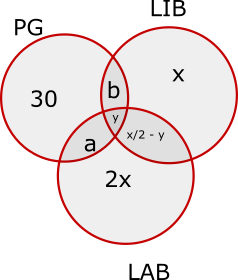### CAT 1991 Question Paper Question 27

Instructions

There were a hundred schools in a town. Of these, the number of schools having a play - ground was 30, and these schools had neither a library nor a laboratory. The number of schools having a laboratory alone was twice the number of those having a library only. The number of schools having a laboratory as well as a library was one-fourth the number of those having a laboratory alone. The number of schools having either a laboratory or a library or both was 35.

Question 27

# What was the ratio of schools having laboratory those having library?

SolutionThe diagram for this question has been shown:

Total number of schools having either or LAB or LIB or both = a+b+x/2 - y + y + 3x = 7x/2 + a + b = 35

It has been given that the schools having playground don't have a Library or Laboratory.

Hence  a = b = y = 0

7x/2 = 35

x = 10

Required ratio = 25:15 = 5:3